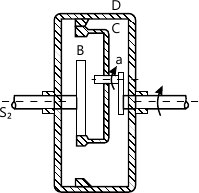MORE IN Theory of Machines - 2
MU Mechanical Engineering (Semester 5)
Theory of Machines - 2
May 2015
Total marks: --
Total time: --
INSTRUCTIONS
(1) Assume appropriate data and state your reasons
(2) Marks are given to the right of every question
(3) Draw neat diagrams wherever necessary

1 (a) Why single plate clutches are dry whereas multi plate clutches are wet?
5 M
1 (b) Prove that sensitiveness of a pro ell governor is greater than that of porter governor.
5 M
1 (c) Explain self-energizing and self-locking effect in block brake.
5 M
1 (d) Explain the necessity of gear box in automobile.
5 M
1 (e) Derive the expression to determine gyroscople couple.
5 M

2 (a) A leather faced conical clutch has a cone angle of 30°. If the intensity of pressure between the contact surfaces is limited to 0.35 N/mm2 and the breadth of the conical surface is not to exceed one-third of the mean radius, find the dimensions of the contact surface to transmit 22.5 KW at 2000RPM. Assuming uniform rate of wear and take coefficient of friction as 0.15.
10 M
2 (b) In case of band and block there are 10 blocks. The coefficient of friction is 0.4. Each block subtends an angle of 16° with the centre of the wheel. The brake absorbs 225 KW at 300RPM. The effective diameter is 80cm. Find the force applied at the end of lever 25 cm long if band is attached at a distance of 15 cm and 3 cm either side of the fulcrum.
10 M

3 (a) In the epicyclic gear train shown in Fig. 1, a gear C which has teeth cut internally and externally is free to rotate on an arm driven by the shaft S1. It meshes externally with the casing D and internally with the pinion B. The gears have following number of teeth TB=24, Tc=32 and Td=48, Find the velocity ratio between
(i) S1 and S2 when D is fixed.
(ii) S1 and D when S2 is fixed. What will be the torque required to fix the casing D if a torque required to fix the casing D if a torque of 300Nm is applied to shaft S1?12 M
3 (b) Derive an expression for 'finding angle of heel' of a two wheeler negotiating a turn.
8 M

4 (a) A horizontal gas engine running at 210 RPM has a bore of 220mm and a stroke of 440mm. The connecting rod is 924 mm long and the reciprocating parts weight 20Kg. When the crank has turned through an angle of 30° from the inner dead centre, gas pressure on the cover and the crank sides are 500KN/m2 and 60KN/m2 respectively. Diameter of the piston rod is 40mm. Determine (i) turning moment on crank shaft. (ii) thrust on the bearing. (iii) acceleration of the flywheel which has a mass of 8 kg and radius of gyration of 600mm while the power of engine is 22Kw.
12 M
4 (b) Single plate clutch transmits 25 KW at 900RPM. The maximum pressure intensity between the plates is 85 KN/m2. The outer diameter of the plate is 360mm. Both sides of the plate are effective and the coefficient of friction is 0.25.
Determine the (i) Inner diameter of the plate
(ii) Axial force to engage the clutch.
8 M

5 (a) The arms of Hartnell governor are of equal length. When the sleeve is in the mid position, the masses rotate in a circle with diameter of 150mm (the arms are vertical in the mid position). Neglecting friction, the equilibrium speed for this position is 360 RPM. Maximum variation of speed taking friction into account, is to be 6% of the mid position speed for a maximum sleeve movement of 30mm. The sleeve mass is 5 kg and the friction of sleeve is 35N.
Assuming that the power of the governor is sufficient to overcome the friction by 1% change of speed on each side of mean position. Find (neglecting obliquity effects of the arms), the (i) mass of each rotating of ball. (ii) spring stiffness. (iii) initial compression of the spring.
12 M
5 (b) State the different types of governors. What is the difference between centrifugal and inertia governors? Why is the former preferred to the later?
8 M

6 (a) Each wheel of four wheeled rear engine automobile has a moment of inertia 2.4 Kg m2 and an effective diameter of 660mm. The rotating parts of the engine have a moment of inertia of 1.2 Kg m2. The gear ratio of engine to the back wheel is 3 to 1. The engine axis is parallel to the rear axle and the crankshaft rotates in the same sense as the road wheels. The mass of vehicle is 2200Kg and the centre of the mass is 550 mm above the road level. The track width of the vehicle is 1.5m. Determine the limiting speed of the vehicle around a curve with 80m radius so that all the four wheels maintain contact with the road surface.
10 M
6 (b) A riveting machine is driven by a constant torque 3 kW motor. The moving parts including the flywheel are equivalent to 150 kg at 0.6 m radius. One riveting operation takes 1 second and absorbs 10000 N-m of energy. The speed of the flywheel is 300 RPM before reveting. Find the speed immediately after riveting. How many rivets can be closed per minute?
10 M

More question papers from Theory of Machines - 2